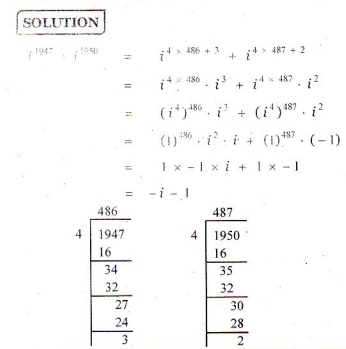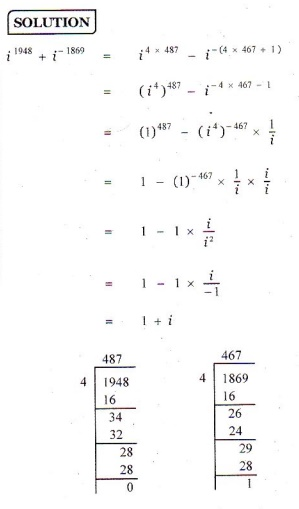Home | | Maths 12th Std | Exercise 2.1: Introduction to Complex Numbers

# Exercise 2.1: Introduction to Complex Numbers

Maths Book back answers and solution for Exercise questions - Mathematics : Complex Numbers: Exercise Problem Questions with Answer, Solution

EXERCISE 2.1

Simplify the following:

1. i 947 + i19502. i 1948 - i 18695. i i2 i3 i20006.1. ŌłÆ1 ŌłÆ

2. 1+ i

3. 0

4. 0

5. 1

6. 1ŌłÆ i

Tags : Problem Questions with Answer, Solution , 12th Mathematics : UNIT 2 : Complex Numbers
Study Material, Lecturing Notes, Assignment, Reference, Wiki description explanation, brief detail
12th Mathematics : UNIT 2 : Complex Numbers : Exercise 2.1: Introduction to Complex Numbers | Problem Questions with Answer, Solution